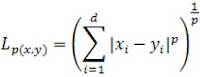Please visit, subscribe and share 10 Minutes Lectures in Computer Science

# Data warehousing and Data mining solved quiz questions and answers, multiple choice questions MCQ in data mining, questions and answers explained in data mining concepts, data warehouse exam questions, data mining mcq

## 1. Minkowski distance is a function used to find the distance between two

a) Binary vectors

b) Boolean-valued vectors

c) Real-valued vectors

d) Categorical vectors

Minkowski distance finds the distance between two real-valued vectors. It is a generalization of the Euclidean and Manhattan distance measures and adds a parameter, called the “order” or “p“, that allows different distance measures to be calculated.

Minkowski distance,### If p=2 then L2 which is Euclidean distance (change p with 2 in above equation)

2. Which of the following distance measure is similar to Simple Matching Coefficient (SMC)?

a) Euclidean distance

b) Hamming distance

c) Jaccard distance

d) Manhattan distance

## Hamming distance is the number of bits that are different between two binary vectors.

The Hamming distance is similar to the SMC in which both methods look at the whole data and looks for when data points are similar and dissimilar.  The Hamming distance gives the number of bits that are different whereas the SMC gives the result of the ratio of how many bits were the same over the entirety of the sample set.  In a nutshell, Hamming distance reveals how many were different, SMC reveals how many were same, and therefore one reveals the inverse information of the other.

SMC = Hamming distance / number of bits

3. The statement “if an itemset is frequent then all of its subsets must also be frequent” describes _________ .

a) Unique item property

b) Downward closure property

c) Apriori property

d) Contrast set learning

Answer: (b) Downward closure property and (c) Apriori property

## The Apriori property state that if an itemset is frequent then all of its subsets must also be frequent.

Apriori algorithm is a classical data mining algorithm used for mining frequent itemsets and learning of relevant association rules over relational databases.

Apriori property expresses monotonic decrease of an evaluation criterion accompanying with the progress of a sequential pattern.

### Both downward closure property and Apriori property are synonyms to each other.

4. Prediction differs from classification in which of the following senses?

a) Not requiring a training phase

b) The type of the outcome value

c) Using unlabeled data instead of labeled data

d) Prediction is about determining a class

Answer: (b) The type of the outcome value

## Predicting class labels is classification, and predicting values (e.g. using regression techniques) is prediction.

Classification is the process of identifying the category or class label of the new observation to which it belongs.  Predication is the process of identifying the missing or unavailable numerical data for a new observation.

5. The statement “if an itemset is infrequent then it’s superset must also be an infrequent set” denotes _______.

a) Maximal frequent set.

b) Border set.

c) Upward closure property.

d) Downward closure property.

 Answer: (c) Upward closure property Any subset of a frequent item set must be frequent (downward closure property) or any superset of an infrequent item set must be infrequent (Upward closure property). Both are Apriori properties.

**********************